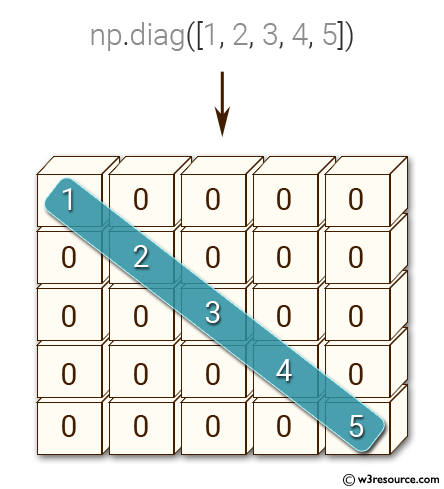﻿ NumPy: Create a 5x5 zero matrix with elements on the main diagonal equal to 1, 2, 3, 4, 5 - w3resource# NumPy: Create a 5x5 zero matrix with elements on the main diagonal equal to 1, 2, 3, 4, 5

## NumPy: Basic Exercise-29 with Solution

Write a NumPy program to create a 5x5 zero matrix with elements on the main diagonal equal to 1, 2, 3, 4, 5.

Sample Solution :

Python Code :

``````import numpy as np
x = np.diag([1, 2, 3, 4, 5])
print(x)
``````

Sample Output:

```[[1 0 0 0 0]
[0 2 0 0 0]
[0 0 3 0 0]
[0 0 0 4 0]
[0 0 0 0 5]]
```

Pictorial Presentation:Python Code Editor:

Have another way to solve this solution? Contribute your code (and comments) through Disqus.

What is the difficulty level of this exercise?

Test your Python skills with w3resource's quiz

﻿

## Python: Tips of the Day

Checks if the given number falls within the given range.

Example:

```def tips_range(n, start, end = 0):
return start <= n <= end if end >= start else end <= n <= start
print(tips_range(2, 4, 6))
print(tips_range(4, 8))
print(tips_range(1, 3, 5))
print(tips_range(1, 3))
```

Output:

```False
True
False
True
```